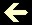PRIME NUMBERS

Prime numbers are the integers, bigger than 1, that haven't other exact divider, except itself and the unity. To check it, we divide the number, one after another, by 2, 3, 5, 7, 9, 11, 13, 15, 17 etc. until we reach a division with remainder zero (and so it isn't a prime number) or until we reach a division with the quotient smaller than the divider and the remainder different of zero (and so it's a prime number).

Input a number and see if it's a prime number or not. If it isn't a prime number, you'll have its 3rd divider:

 Number Prime? 3rd divider